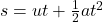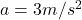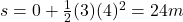a car accelerates from rest at 3 m / s^2 along a straight road. how far has the car travelled after 4 s

Question

a car accelerates from rest at 3 m / s^2 along a straight road. how far has the car travelled after 4 s

in progress 0
5 months 2021-08-12T23:02:53+00:00 1 Answers 1 views 0

24 m

Explanation:

The motion of the car is a uniformly accelerated motion (=at constant acceleration), therefore we  can find the distance covered by using the following suvat equation:where

s is the distance covered

u is the initial velocity

t is the time elapsed

a is the acceleration

For the car in this problem:

u = 0, since the car starts from restis the acceleration

t = 4 s is the time elapsed

Therefore, the distance covered is: